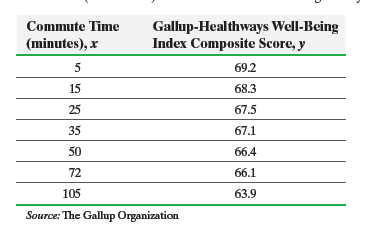×
Get Full Access to Statistics: Informed Decisions Using Data - 5 Edition - Chapter 14.1 - Problem 11
Get Full Access to Statistics: Informed Decisions Using Data - 5 Edition - Chapter 14.1 - Problem 11

×

# ?An Unhealthy Commute The following data represent commute times (in minutes) and a score on a well-being survey. Use the results from PISBN: 9780134133539 240

## Solution for problem 11 Chapter 14.1

Statistics: Informed Decisions Using Data | 5th Edition

• Textbook Solutions
• 2901 Step-by-step solutions solved by professors and subject experts
• Get 24/7 help from StudySoup virtual teaching assistantsStatistics: Informed Decisions Using Data | 5th Edition

4 5 1 295 Reviews
31
0
Problem 11

An Unhealthy Commute The following data represent commute times (in minutes) and a score on a well-being survey.Use the results from Problem 17 in Section 4.2 to answer the following questions:

(a) Treating commute time as the explanatory variable, x,determine the estimates of0 and1.

(b) Compute the standard error of the estimate, Se.

(c) Determine Sb1.

(d) A normal probability plot of the residuals indicates it is reasonable to conclude the residuals are normally distributed. Test whether a linear relation exists between commute time and well-being index composite score at the a = 0.05 level of significance.

(e) Construct a 95% confidence interval about the slope of the true least-squares regression line.

Step-by-Step Solution:

Step 1 of 5) An Unhealthy Commute The following data represent commute times (in minutes) and a score on a well-being survey.Use the results from Problem 17 in Section 4.2 to answer the following questions: (a) Treating commute time as the explanatory variable, x, determine the estimates of 0 and 1. (b) Compute the standard error of the estimate, Se. (c) Determine Sb1. (d) A normal probability plot of the residuals indicates it is reasonable to conclude the residuals are normally distributed. Test whether a linear relation exists between commute time and well-being index composite score at the a = 0.05 level of significance. (e) Construct a 95% confidence interval about the slope of the true least-squares regression line. Summary In this chapter, we introduced probability models for random variables. A random variable represents the numerical measurement of the outcome from a probability experiment.

Step 2 of 2

##### ISBN: 9780134133539

The full step-by-step solution to problem: 11 from chapter: 14.1 was answered by , our top Statistics solution expert on 01/15/18, 03:19PM. The answer to “?An Unhealthy Commute The following data represent commute times (in minutes) and a score on a well-being survey. Use the results from in Section 4.2 to answer the following questions:(a) Treating commute time as the explanatory variable, x, determine the estimates of 0 and 1.(b) Compute the standard error of the estimate, Se.(c) Determine Sb1.(d) A normal probability plot of the residuals indicates it is reasonable to conclude the residuals are normally distributed. Test whether a linear relation exists between commute time and well-being index composite score at the a = 0.05 level of significance.(e) Construct a 95% confidence interval about the slope of the true least-squares regression line.” is broken down into a number of easy to follow steps, and 109 words. Statistics: Informed Decisions Using Data was written by and is associated to the ISBN: 9780134133539. This textbook survival guide was created for the textbook: Statistics: Informed Decisions Using Data, edition: 5. Since the solution to 11 from 14.1 chapter was answered, more than 218 students have viewed the full step-by-step answer. This full solution covers the following key subjects: . This expansive textbook survival guide covers 88 chapters, and 2422 solutions.

## Discover and learn what students are asking

Statistics: Informed Decisions Using Data : The Design of Experiments
?What is replication in an experiment?

Statistics: Informed Decisions Using Data : Tests for Independence and the Homogeneity of Proportions
?The following table contains the number of successes and failures for three categories of a variable. Test whether the proportions are

Unlock Textbook Solution